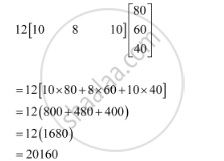Share

# The Bookshop of a Particular School Has 10 Dozen Chemistry Books, 8 Dozen Physics Books, 10 Dozen Economics Books. Their Selling Prices Are Rs 80, Rs 60 and Rs 40 Each Respectively. Find the Total Amount the Bookshop Will Receive from Selling All the Books Using Matrix Algebra. - CBSE (Science) Class 12 - Mathematics

#### Question

The bookshop of a particular school has 10 dozen chemistry books, 8 dozen physics books, 10 dozen economics books. Their selling prices are Rs 80, Rs 60 and Rs 40 each respectively. Find the total amount the bookshop will receive from selling all the books using matrix algebra.

#### Solution

The bookshop has 10 dozen chemistry books, 8 dozen physics books, and 10 dozen economics books.

The selling prices of a chemistry book, a physics book, and an economics book are respectively given as Rs 80, Rs 60, and Rs 40.

The total amount of money that will be received from the sale of all these books can be represented in the form of a matrix as:Thus, the bookshop will receive Rs 20160 from the sale of all these books.

Is there an error in this question or solution?

#### Video TutorialsVIEW ALL 

Solution The Bookshop of a Particular School Has 10 Dozen Chemistry Books, 8 Dozen Physics Books, 10 Dozen Economics Books. Their Selling Prices Are Rs 80, Rs 60 and Rs 40 Each Respectively. Find the Total Amount the Bookshop Will Receive from Selling All the Books Using Matrix Algebra. Concept: Introduction of Matrices.
S